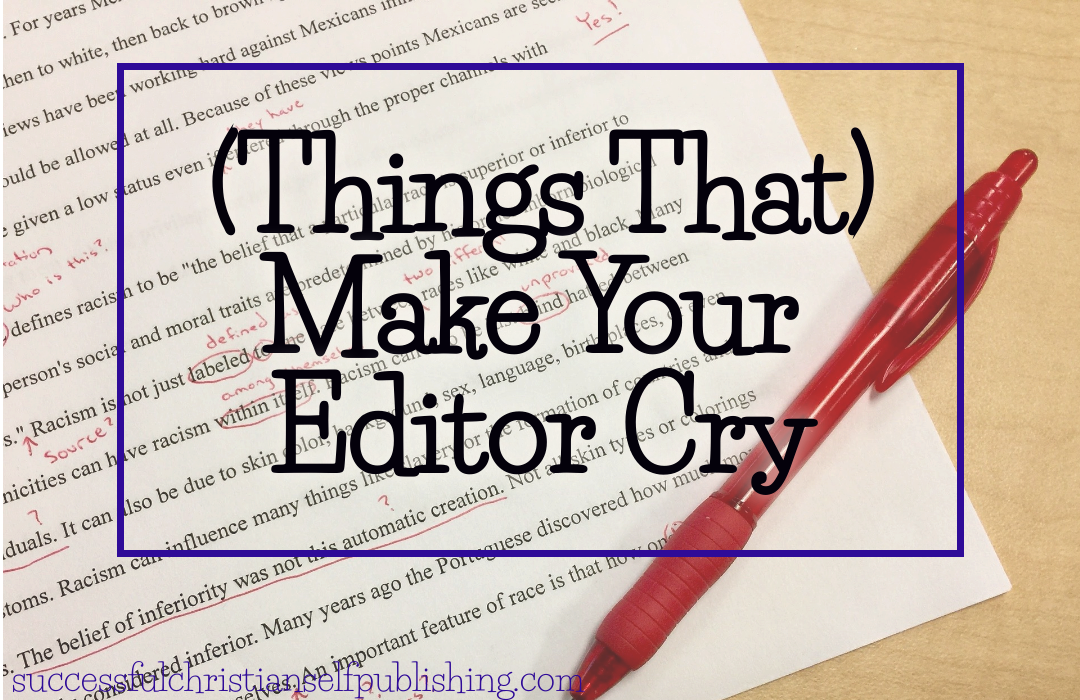# Make Your Editor Cry: Formula (Awkward Plural)BLUF: (Bottom Line Up Front)
The plural of formula is formulae or less commonly formulas.

The definition of formula: a customary or set form or method allowing little room for originality

The singular of formula is formula. The plural of formula is formulae or formulas. You can use either, just be consistent throughout the entire text. The noun formula has a Latin root—diminutive of forma form—which is the derivation of the plural formulae. The plural formulae does not conform to the standard rules for forming the plurals of nouns in English.

Thanks to common informal usage, formulas, which conforms to the standard rules for forming plurals, is also now acceptable, though I would avoid using it in narrative text. It could be used in dialogue to demonstrate a character who doesn’t know better.

```Formulas are applied to provide a mathematical solution for real world problems. Formulae are used to express relationships between various quantities.```